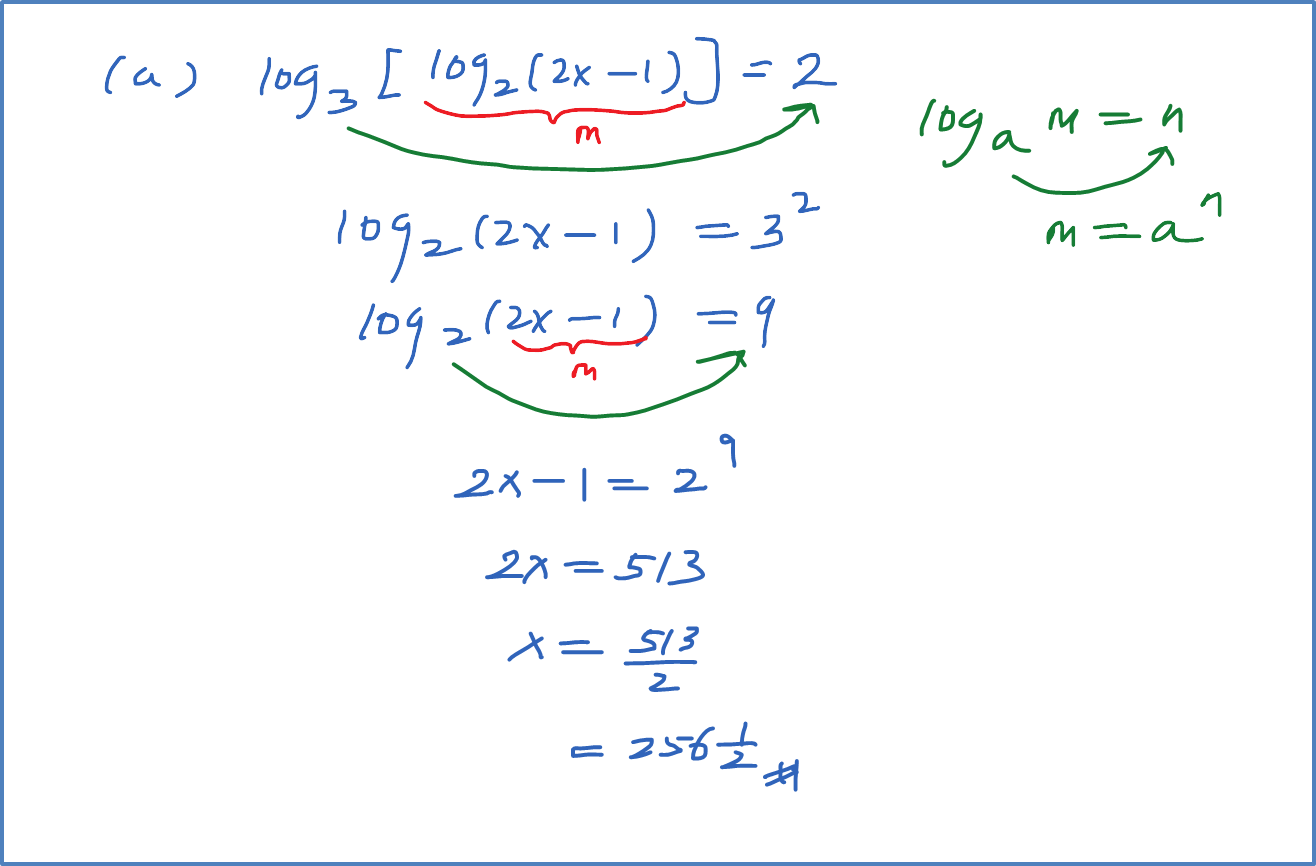# 4.4.3 Logarithms Equation – Example 3

Example 3
Sole the following equation:
(a) ${\mathrm{log}}_{3}\left[{\mathrm{log}}_{2}\left(2x-1\right)\right]=2$
(b) ${\mathrm{log}}_{16}\left[{\mathrm{log}}_{2}\left(5x-4\right)\right]={\mathrm{log}}_{9}\sqrt{3}$